# GEF3D Run Examples

This document briefly describes how to run the GEF3D examples. Please also read the install instruction (GEF3D Installation) and have a look at the list of supported graphics cards (GEF3D List of Graphics Card).

GEF3D provides three examples:

1. a simple graph editor for drawing a graph with nodes and edges
2. a "3D-fied" version of the Ecore tools diagram editor
3. a "3D-fied" version of the UML2 tools activity, class, and use case diagram editors

## Setup

As GEF3D can only be "installed" by importing the sources from the subversion repository, the examples are to be run in a so called runtime instance. Note that is doesn't matter which "project" you select, as usually all projects in the workspace are activated in the runtime instance. Figure 1 shows the context menu to start the runtime instance.

Sometimes it is necessary to configure the runtime configuration. In the "Run configurations.." menu you can set some runtime parameters, as shown in Figure 2.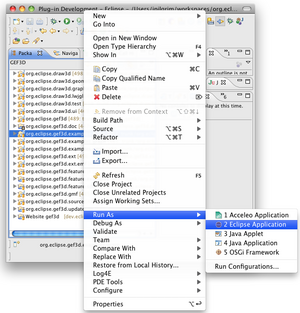Figure 1: Run As Eclipse Application

On Mac OS X, you have to add a VM argument "-d32" if you are running Eclipse 32bit. In general, you maybe have to increase the so called "permsize" setting (-XX:PermSize=128m -XX:MaxPermSize=512m). In general, it is a good idea to adust the heap size (-Xmx512m).

## Graph Example

In the runtime instance, create a new project. Then you can create a new graph example by using the GEF3D example wizard, see Figure 3.

You can set several options (number of nodes and edges, number of planes) and finally open the diagram. You may have to refresh the project in the package explorer (F5) in order to see the created diagram file. In general, you can select which editor to use in order to open the file, as shown in Figure 4. This is not only true for the graph example, but for Ecore or UML diagrams as well. Actually, this is one of the benefits of GEF3D: You can open the very same diagrams in 2D and 3D!

Figure 5 shows a simple graph opened with the 3D editor. This is the simplest example, and if you cannot open a simmple graph with the 3D editor, then you probably have some more complicated OpenGL problems.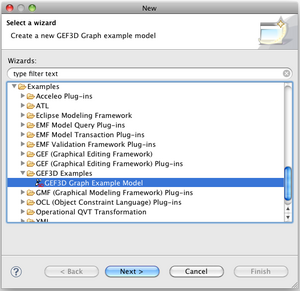Figure 3: Graph example wizard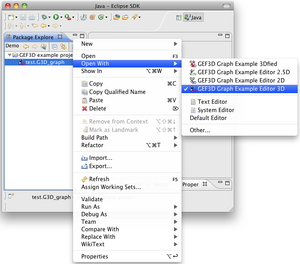Figure 4: Graph example, context menu "Open With..."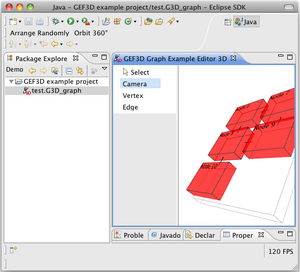Figure 5: Sample graph opened with 3D editor

## Ecore Diagram Example

In order to open an ecore diagram with the 3D ecore editor, you firstly have to create a new diagram using the original Ecore tools wizard, as shown in Figure 6.

Pay attention to really use the Ecore tools wizard and not some other ecore diagram editor. For the example, use the default file name, the diagram is opened with the 2D editor by default (see Figure 7). Create a new class and save the diagram. This step is necessary as the 3D editor lacks the functionality to open an empty diagram.

Close the 2D editor and open the very same diagram with the "Ecore Tools Editor 3D" as shown in Figure 8. Actually, the "ecorediagram" is opened, not the ecore file itself!

If everything works correctly, you should be able to open the diagram with the 3D ecore editor, as shown in Figure 9. In general, you should be able to edit the ecore diagram in 3D, just as if you have opened it in the 2D editor. Howevery, there may be some bugs in the 3D version.Figure 7: Edit ecore diagram with 2D version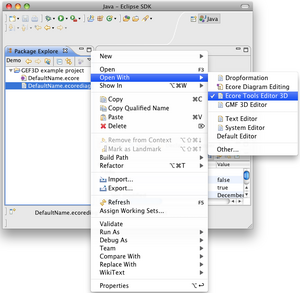Figure 8: Context menu of ecore diagram file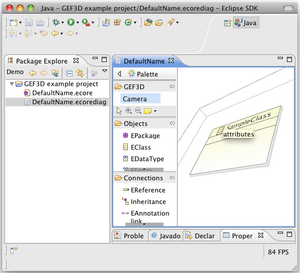Figure 9: Ecore diagram opened with 3D version

If you have problems opening the examples, post a message to the GEF3D newsgroup. It always helps if we know which graphics card you are using, if the LWJGL test view is running, and what error messages (maybe with stack trace) were displayed.